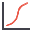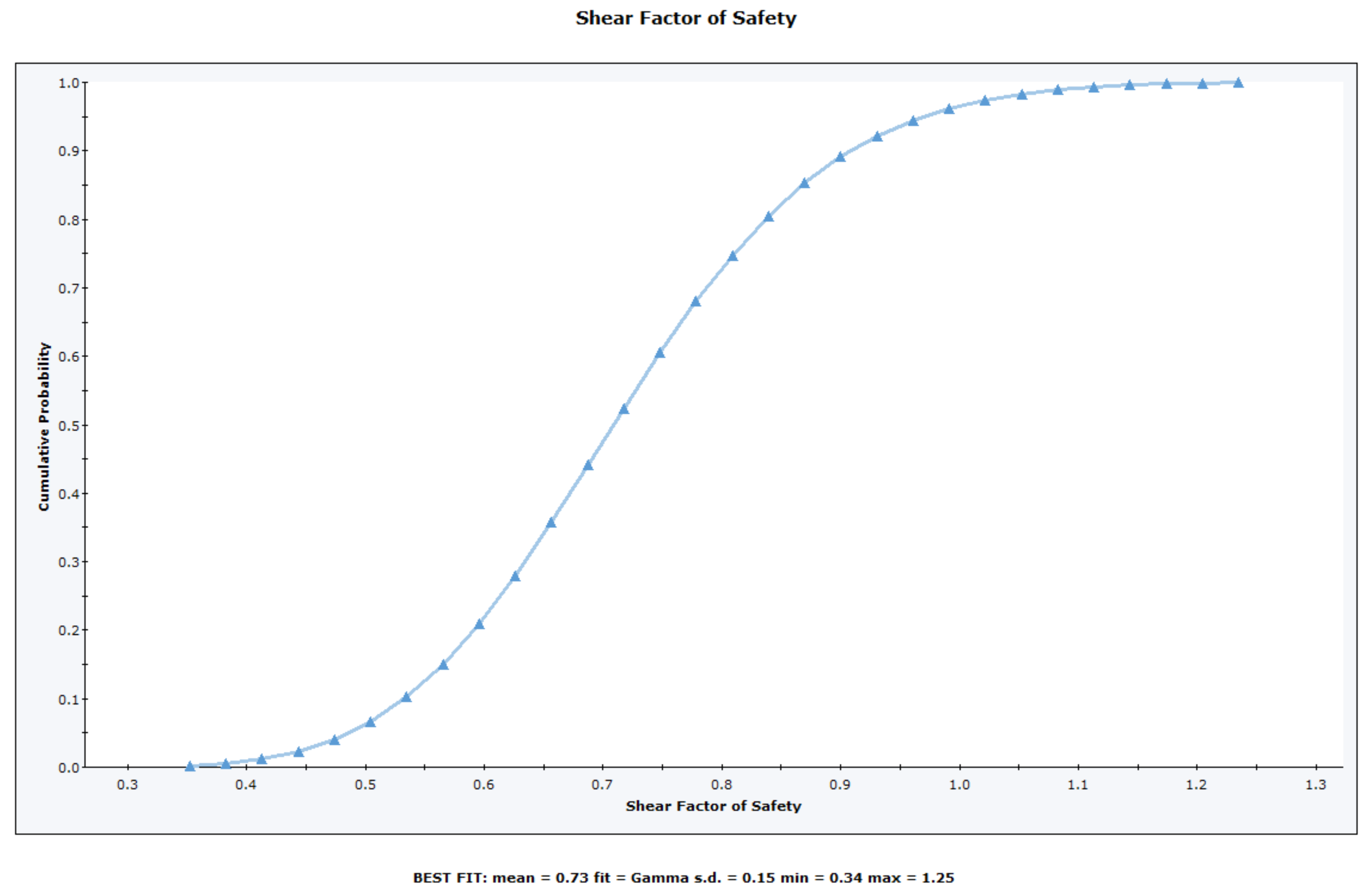The Rocscience International Conference 2021 Proceedings are now available. Read Now

# Cumulative Plot

To plot a Cumulative Distribution after a Probabilistic Analysis:

1. Select Statistics > Plot Cumulative or the "Plot Cumulative"toolbar button.
2. Select the Failure Mode (e.g. Shear, Elastic Buckling, Arch Snap Thru, or Compression, whichever is applicable).
3. Select the Data to Plot (e.g. Factor of Safety, or one of your input data random variables).
4. Enter a Number of Bins. The default is 30, but any value between 2 and 100 can be entered.
5. Select Plot.

The Cumulative Distribution will be generated, and the mean, standard deviation, minimum and maximum values for the data plotted, will be listed at the bottom of the plot.

A Cumulative Distribution is, mathematically speaking, the integral of the normalized probability density function. Practically speaking, a point on the cumulative distribution gives us the probability that a random variable will be LESS THAN OR EQUAL TO a specified value.

Simply put, if (X , Y) is a point on the cumulative distribution S-curve, then Y = the probability that the random variable will be <= X.

The data Sampler allows you to obtain the coordinates of points on the S-curve.

The same Data Types, which can be plotted with Plot Histogram, can also be plotted as Cumulative Distributions – see the Data Type topic for details.

Cumulative plot of safety factor distributionSeveral options are available in the right-click menu for Cumulative Distributions, including: